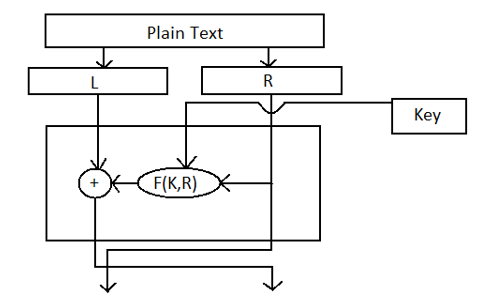# Feistel Cipher in Cryptography

In this tutorial, we will learn about the Feistel Cipher which is the structure used to create block cipher. Here, we will be studying the Feistel structure first, then about where this structure is implemented and finally, how the encryption of the data is done in the Feistel Cipher technique? By Monika Sharma Last updated : May 24, 2023

## What is Feistel Cipher in Cryptography?

The Feistel Cipher is a structure used to create block ciphers. It has many rounds of encryption to increase security. In each round, different techniques are applied to the plain text to encrypt it. Each round has one substitution technique. The plain text after passing through all these rounds gets converted into the Ciphertext. The complete process of the encryption is explained as follows,

## The Feistel Cipher Encryption Process

The process of encryption Feistel Cipher takes place as follows,

1. In this Cipher, the plain text is divided into two equal parts. The left part is denoted as L and the Right part is denoted as R.
2. Every round has an encryption function that is applied to the plain text. (It is applied only to one of the two divisions of the plain text, that is to the left one.)
3. The encryption function is applied on the left part of the plain text and the right part goes unchanged in every round.
4. The encryption function has two parameters: Encryption key and Right part of the plain text.
5. XOR operation is performed between the Left part and the encryption function.
6. The Right part becomes the Left part of the next round and the output of the XOR operation becomes the Right part of the next round. It means that the substituted right part and unchanged right part are swapped for the next round.
7. Each round has a different encryption key or we can say that the key is round dependent, i.e. the key for every round is generated in advance.
8. The process shown above is of a single round. The number of rounds depends upon the algorithm of the process.

The difficult part of this algorithm is designing the round function because it must be applied in every round until the final ciphertext is received. The more the number of rounds, the more secure the data becomes.The process of one round is shown in the diagram

## Decryption Process of Feistel Cipher

The decryption process of Feistel Cipher is given below,

The decryption process of Feistel Cipher is almost the same as the encryption process. Just like we entered the plain text in the Feistel block, we have to do the same with the ciphertext. The ciphertext will be divided into two parts just like the plain text. The only difference is that the keys will be used in reverse order.

## Number of Rounds

The number of rounds depends upon how much security you want. Security is directly proportional to the number of rounds. But simultaneously it slows down the speed of encryption and decryption. The larger the number of rounds is, the creation of ciphertext from plain text and plain text from ciphertext will be slow.